## Spring Design

Design information for engineers, such as spring calculation formulas,

which are the basis of spring design, can be found here.

### Meaning of Symbols

The symbols used for spring design are shown in Table 1 below. The values of the transverse elastic modulus (G) are based on Table 2.

Table 1. Symbols and units used in calculations

Symbol Meaning of Symbol Unit
P Load applied to the spring N
δ Spring deflection mm
Hf Free height mm
Na Number of active coils
R1 Radius of the smallest active coil mm
R2 Radius of The largest active coil mm
G Transverse elastic modulus N/mm2

Table 2.Transverse elastic modulus：G（N/m㎡）

Material G Value
Spring steel material 7.85×104
Stainless Steel

SUS304

SUS316

SUS631J1

6.85×104
6.85×104
7.35×104
Phosphor bronze wire 4.2×104
Beryllium copper wire 4.4×104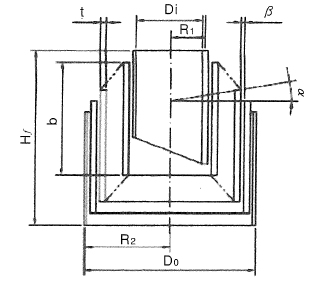### Basic Calculation Formula Used for Design

To calculate the spring constant up to the point where the horizontal spacing between adjacent plates becomes 0 between the plates during compression, the basic formula below can be used.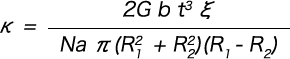To calculate the spring characteristics between the plates after being compressed to the point where the horizontal spacing between adjacent plates becomes 0, the basic formula below can be used.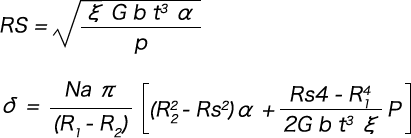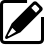Product information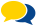Popular questions